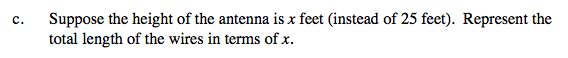### Home > A2C > Chapter Ch11 > Lesson 11.2.1 > Problem11-46

11-46.
1.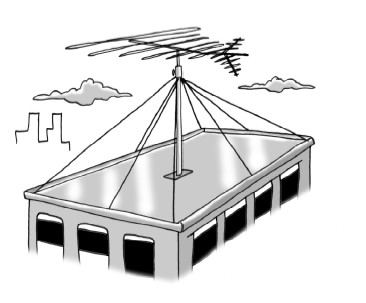The Flat Building's roof is 32 feet wide and 60 feet long. An antenna rises 25 feet above the center of the roof, and wires connect from the top of the antenna to each corner of the roof and to the midpoint of each edge, as shown in the picture below. Homework Help ✎

1. What is the total length of wire needed (without counting any extra needed for attaching it)?

2. The wires that attach to the corners of the building form an angle with the roof. Find the measure of that angle.

3. Suppose the height of the antenna is x feet (instead of 25 feet). Represent the total length of the wires in terms of x.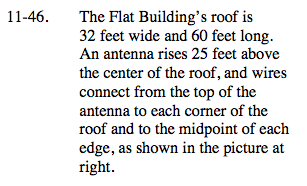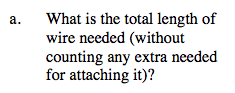306.3 feet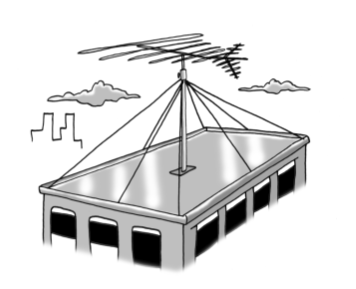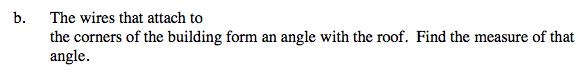Use the trigonometric relationships: